[MyHosting.com]   [KO4BB Home Page]   [Manuals Home Page]   [KO4BB Wiki]

# Differences

This shows you the differences between two versions of the page.

 — precision_timing:lpro_disciplining_with_thunderbolt [2013/01/08 19:00] (current) Line 1: Line 1: + ===== LPRO disciplining with Thunderbolt ===== + + // From the [[Time Nuts Mailing List]]: + // + + In response to request on how I set up my Tbolt with a long time constant to directly discipline a LPRO 101 Rubidium; + + I use a standard LPRO 101 Rb as an external replacement for the Tbolt'​s internal OCXO, + and connect the LPRO's external C_Field adjustment input to the Tbolt'​s Dac out thru a 1K ohm resistor (added as protection for the Tbolt.) + + Due to Tbolt'​s software limitations,​ an Extended_TC setting method needs to be used for setting Time Constants above 1000 sec. + + To calculate the Tbolt'​s Effective TC setting using this extended TC setting method: + + - The Effective_TC of the TBolt is Actual_TC setting times the Multiplier_Factor + - The Effective_Damping factor of a Tbolt is Actual_Damping setting divided by the square root of the Multiplier_Factor + - The Multiplier_Factor is equal to "​Dac_Gain used / Actual_EFC_Gain"​ + + + **Example for setting Tbolt'​s Effective_TC = 20,000 sec, Effective_Damping factor = 0.6 for a LPRO.** + + - Use Multiplier_Factor = 100 + - Dac Gain = +0.90 Hz/V + - TC = 200 sec + - Damping = 6.0 + - Set Initial Dac value to +2.5V, + + After adjusting ​ the LPRO's to 10 MHz using it's internal freq C field pot (with the external C_Field input floating ​ at 2.5V), it is a good idea to limit the Tbolt'​s Dac output swing by setting the Max_Dac out to + 3.0 V, and the Min_Dac out to + 2.0 V. + + + **Example of setting Tbolt'​s Effective_TC = 5,000 sec,  Effective_Damping factor = 0.7** + + (I use this setting to discipline a very stable external dual oven HP 10811.) + + - Use Multiplier factor = 10 + - Dac Gain = -3.24 Hz/V    (Actual Gain for this 10811 is -0.324 Hz/V) + - TC = 500 sec + - Damping = 2.2 + + The effective TC can be measured by how long of time that it takes for a Freq offset error to return to zero, best measured by using LH's Dac voltage plot. + + The effective Damping can be calculated by how much Freq error overshoots there is, which in turn determines how long of time the Phase error takes to return to zero. + + For a damping =  0.6  the Freq overshoot is 33% and Phase correction time is about 3.5 times the Freq correction time  (10%  phase overshoot) + + Using a damping of  0.7,  the Freq overshoot is 25%, & phase correction time is  5x the TC setting. (no phase overshoot) + + With a damping factor of 1.0, there will be very little Freq overshoot, but that causes the phase correction time to be very long. (overdamped Phase response) + + Using Lady Heather, the effective TC and Damping_Factor settings can be verified by plotting the offset freq recovery time to an intentional Dac step error: + + - Disable the control loop (D D) + - Change the Dac voltage (D S ... ) by an amount equal to 1e-10 or 1e-9 (verify on Osc plot) + - Enable the control loop (D E) + - Plot the Phase, Dac_Voltage and Osc_Freq over at least 5 times the effective TC setting. + + Before replacing a Tbolt'​s internal Osc with a cheap rubidium, one should understand the noise tradeoffs, especially at low taus. + Lots of plots available on request + + ws

precision_timing/lpro_disciplining_with_thunderbolt.txt · Last modified: 2013/01/08 19:00 (external edit)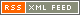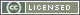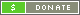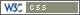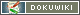##### Except as noted, this entire site Copyright © 2002-2017. KO4BB All rights reserved.